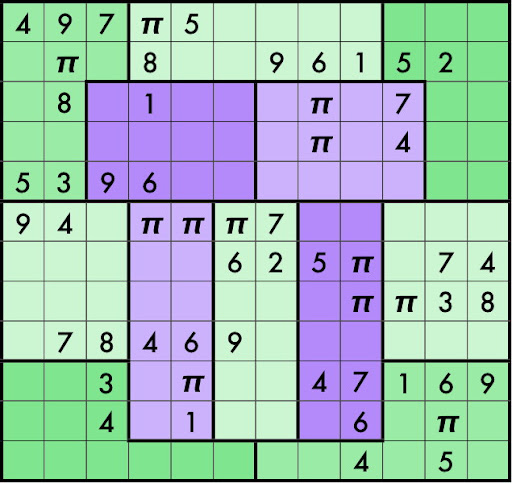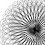### Pi Day and the BBP formula

In the last few days I must write the 47th italian Carnival of Mathematics (Google translation), so I cannot write something about Pi Day. So, in the end of the day, I try to write a little post about this day.$\pi$ is one of the irrational numbers: we cannot write an irrational numbers like a ratio between twointeger numbers, so Pitagora's team called them irrational and hidden their existence. We can define $\pi$ like the ratio between circle and his diameter, and it's impossible that they are contemporary integer. This assertion was proofed in 1761 by Johann Heinrich Lambert, while in 1882 Ferdinand von Lindemann proofed that $\pi$ is a trascendental number, where a trascendental number is a number that we cannot calculate with usual algebric operations.
In ancient times, the first mathematician who evaluate $\pi$ was Archimedes, who used the method of exhaustion, that is a method to evaluate the area of a shape by inscribing inside it a series of polygons that converge to area's shape. In this way Archimedes find $\pi = \frac{211875}{67441} = 3.14163 \cdots$
Today we know 5 triolions of digits, thanks to the work of Shigeru Kondo, who modify a usual home pc in order to perform the calculations using the softwerdeveloped by Alexander Yee. They design an internet site to describe methods, software and results.
Following David Bailey and Jonathan Borwein(1), I find some others interesting site about the calculations of $\pi$'s digits (Inverse Symbolic Calculator, Electronic Geometry Models). Other links at Experimental mathematics. But... what is experimental mathematics?
(...) namely the utilization of modern computer technology as an active tool in mathematical research.(1)
For example using the PSLQ algorithm(2) in 1995 was found the BBP formula for $\pi$: $\pi = \sum_{k=0}^\infty \frac{1}{16^k} \left ( \frac{4}{8k+1} - \frac{2}{8k+4} - \frac{1}{8k+5} - \frac{1}{8k+6} \right )$
This formula permits one to directly calculate binary or hexadecimal digits beginning at the $n$-th digit, without needing to calculate any of the first $n − 1$ digits, using a simple scheme that requires very little memory and no multiple-precision arithmetic software.(1)
You can find others BBP formulas in the David Bailey's compendium (pdf).
(1) Jonathan M. Borwein, David H. Bailey. Experimental Mathematics: Examples, Methods and Implications. Notices of the American Mathematical Society, vol.52, 5, (2005)
(2) PSLQ algorithm, which, compared with other integer relation algorithms in the literature, features superior performance and excellent numerical stability, will produce lower bounds on the (Frobenius) norm of any possible relation for $x$, where $x$ is an arbitrary vector defined on some number field.
Ferguson, H., Bailey, D., & Arno, S. (1999). Analysis of PSLQ, an integer relation finding algorithm Mathematics of Computation, 68 (225), 351-370 DOI: 10.1090/S0025-5718-99-00995-3
Image source: Brainfreeze Puzzles

1.What about that strange Sudoku pattern?

2.The rules are in the original link:
Fill in the grid so that each row, column, and jigsaw region contains 1-9 exactly once and π three times.

http://brainfreezepuzzles.com/main/piday2009.html

1.Ah, thanks, Gianluigi!

Markup Key:
- <b>bold</b> = bold
- <i>italic</i> = italic
- <a href="http://www.fieldofscience.com/">FoS</a> = FoS